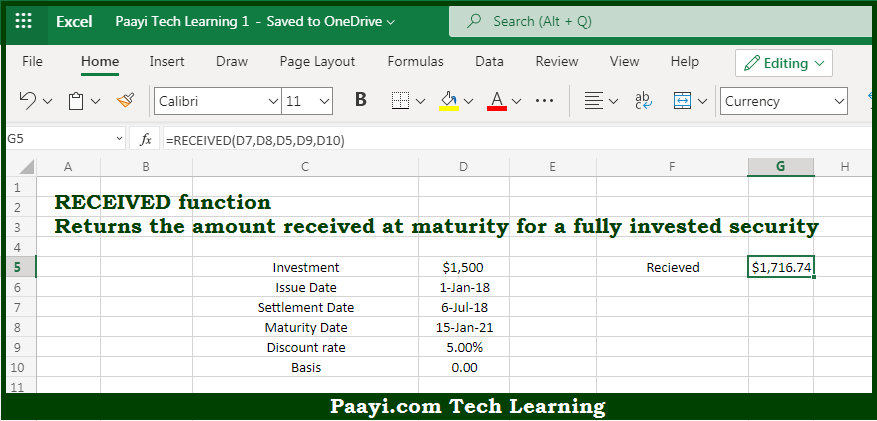# Learn How to Use Microsoft Excel RECEIVED Function

Written by | 0 Comments | 507 Views

In this article, you will learn how to use the Microsoft Excel RECEIVED function and its prime function in Microsoft Excel. You will also get to know the Microsoft Excel RECEIVED function return value and syntax with the help of some examples.

The main purpose of the Microsoft Excel RECEIVED function is to get the amount to be received at maturity. That implies, with the help of the RECEIVED function you can able to return the amount received at maturity for a fully invested security. So, with the help of the RECEIVED function, you can able to get the amount to be received at maturity.

The return value will be the amount received.

Where the arguments:

• settlement: This is the settlement date of the security.
• maturity: This is the maturity date of the security.
• investment: This is the amount invested in the security.
• discount: This is the discount rate of the security.
• basis: This is the day count basis, and the default is 0 (optional).

How to Use Microsoft Excel RECEIVED Function?So we know that Microsoft Excel RECEIVED function you can able to get the amount to be received at maturity. That implies, with the help of the RECEIVED function you can able to return the amount received at maturity for a fully invested security. So, with the help of the RECEIVED function, you can able to get the amount to be received at maturity.